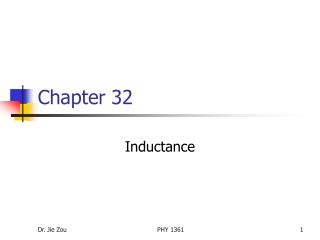DownloadDownload PresentationChapter 32

# Chapter 32

Download Presentation## Chapter 32

- - - - - - - - - - - - - - - - - - - - - - - - - - - E N D - - - - - - - - - - - - - - - - - - - - - - - - - - -
##### Presentation Transcript

1. Chapter 32 Inductance PHY 1361

2. Outline • Self-inductance (32.1) • Mutual induction (32.4) • RL circuits (32.2) • Energy in a magnetic field (32.3) • Oscillations in an LC circuit (32.5) • The RLC circuit (32.6, 33.5) PHY 1361

3. Self inductance • Self induction: the changing flux through the circuit and the resultant induced emf arise from the circuit itself. The emf L set up in this case is called a self-induced emf. • L = -L(dI/dt) • L = - L/(dI/dt): Inductance is a measure of the opposition to a change in current. • Inductance of an N-turn coil: L = NB/I; SI unit: henry (H). Due to self-induction, the current in the circuit does not jump from zero to its maximum value instantaneously when the switch is thrown closed. PHY 1361

4. Mutual induction • Mutual induction: Very often, the magnetic flux through the area enclosed by a circuit varies with time because of time-varying currents in nearby circuits. This condition induces an emf through a process known as mutual induction. • An application: An electric toothbrush uses the mutual induction of solenoids as part of its battery-charging system. PHY 1361

5. RL circuits • An inductor: A circuit element that has a large self-inductance is called an inductor. • An inductor in a circuit opposes changes in the current in that circuit. • A RL circuit: • Kirchhoff’s rule: • Solving for I: I = (/R)(1 – e-t/) •  = L/R: time constant of the RL circuit. • If L  0, i.e. removing the inductance from the circuit, I reaches maximum value (final equilibrium value) /R instantaneously. PHY 1361

6. Energy in a magnetic field • Energy stored in an inductor: U = (1/2)LI2. • This expression represents the energy stored in the magnetic field of the inductor when the current is I. • Magnetic energy density: uB = B2/20 • The energy density is proportional to the square of the field magnitude. PHY 1361

7. Oscillations in an LC circuit • Total energy of the circuit: U = UC + UL = Q2/2C + (1/2)LI2. • If the LC circuit is resistanceless and non-radiating, the total energy of the circuit must remain constant in time: dU/dt = 0. • We obtain • Solving for Q: Q = Qmaxcos(t + ) • Solving for I: I = dQ/dt = - Qmaxsin(t + ) • Natural frequency of oscillation of the LC circuit: PHY 1361

8. The RLC circuit • The rate of energy transformation to internal energy within a resistor: dU/dt = - I2R • Equation for Q: • Compare this with the equation of motion for a damped block-spring system: • Solving for Q: Q = Qmaxe-Rt/2Lcos(dt) PHY 1361